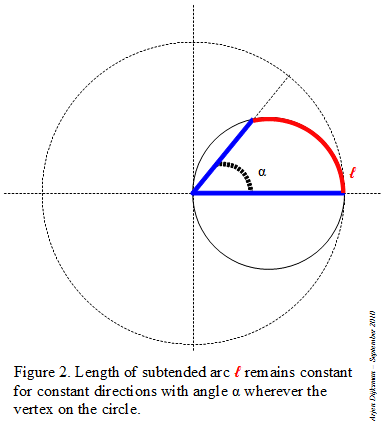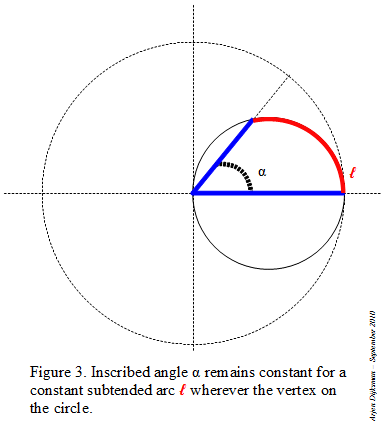## Sunday, March 27, 2011

### Morley triangle derived from the tripling of an angleMorley equilateral triangle at the intersection of the trisectors (from Wikimedia Commons)
In a previous post, I mentioned Morley's miracle: an equilateral triangle appearing at the points of intersection of the angle trisectors of any triangle.

Frank Morley was an excellent teacher and chess player (see some more about him at Pat'sBlog). If he had lived today, I like to think of him having his own blog communicating about his passion for math and chess, submitting his recreational problems. If he hadn't discovered the equilateral triangle at the intersection of trisectors, it's probable that this theorem would still be unknown. Trisection of angles is a controversial subject for research, especially among academia, where it is associated with suspicion of crankiness (see Underwood Dudley's book about trisectors). A pity... because trying to understand how to divide angles is a "natural" question, of which we shouldn't be ashamed, provided that we modestly take into account what has been found by other people.

All the proofs of Morley's theorem that I know of start with a result: an equilateral triangle or a triangle already drawn with its trisectors (see the different proofs referenced on the very complete French site Abracadabri or at the end of Alexander Bogolmony's Cut the Knot site). In this sense, they are backward proofs, which keeps some mystery about the physical origin of the equilateral triangle. I tried something different, a bit similar in spirit to my Archimedes tripling circle: How can we multiply an angle by 3, from which would result a Morley triangle embedded in a triangle of any shape? So here follows an alternative forward proof for Morley's theorem.

Step 1: I start with a circle in which I inscribe an angle α inferior to 60°. The lines are intercepting an arc on the circle.

Step 2: I duplicate the angle by tracing a little circle centered at one of the endpoints of the arc and with radius the chord of that arc. I repeat the same operation to triplicate the angle (see Figure 1).
Step 3: When two circles of equal size and common radius intersect, two equilateral triangles appear, so I draw both of them: Figure 2.
Step 4: I draw supplementary equilateral triangles at both sides, with the help of the intersections of the circles and the outer lines of the triplicate angle, see Figure 3.
Step 5: The magenta colored equilateral triangle is the Morley triangle of the arbitrary triangle we are looking for. The Morley triangle is a pivotal triangle, so the other vertices of the arbitrary triangle can be found through symmetric construction of the initial inscribing circle centered towards the other sides of the Morley triangle, see the red circles at Figure 4.
Step 6: I complete the figure with the triplicated angles β and γ in the red inscribing circles. The sides of the searched triangle are the outer lines of the triplicated angles, see Figure 5.
With this tripling angle construction, we always obtain an equilateral triangle at the intersection of the trisectors of a triangle. When the initial vertex is moved on the initial inscribing circle, all triangle shapes can be generated for any initial angle α between 0 and 60°, which proves the Morley theorem for any triangle.

## Monday, March 14, 2011

### Archimedes angle trisection or tripling?

Pi-day today, so a nice occasion to write something about circles. π is the ratio between the circles' perimeter (περίμετρος in Greek) and diameter.

In the previous post, I explored some angular tricks using circles. The circle is a key geometrical object when dealing with angles. For example, Archimedes' construction to trisect an angle uses a circle and a straight line with a marked unit. But for clarity's sake, I prefer to draw a set of circles which show how a straight line can mark odd multiples of a unit angle α on intersecting circles, see Figure 1. In that way, it is easier to see how Archimedes' trisection circle relates to the multiplication and division of angles by odd integers, see alternative Figure 2.

This makes me think that Archimedes' angle trisection is in fact the inverse of "Archimedes' angle tripling".

## Wednesday, March 9, 2011

### Playing with angles

A few months ago, I prepared this post but didn't feel ready to publish it. It raised more questions than it answered, so I wanted to have a little more background about it. Hopeless wish! There's so much about angles that I don't fully understand. With pi-day approaching, I'm also looking for some inspiration about circles and angles, so I thought publishing might get me in the direction...

I really became aware of the difficulty to divide an angle into three equal angles (a.k.a. angle trisection) some three years ago. It's bloody hard to find general procedures to divide precisely a circular arc into three equal arcs. There are some tricks that are well documented on the web, but you won’t find a simple geometric procedure using only a compass and an unmarked ruler. It has even been "proven" impossible. We only know of some "cheating" tricks like using a marked ruler (for Archimedes' trisection), origami or mechanical tools that will allow you to trisect precisely an angle. And there is an equilateral triangle that appears "by miracle" when you trisect all angles of a triangle, a result found by mathematician Frank Morley (because he himself tried to better understand or crack that impossibility?). So the angle trisection really became to intrigue me and I progressively dug into it.One of the questions that has obsessed me is : How do we characterize an “Angle”? I’ve already written a post on that question, pointing out the utility of the chord subtending the circular arc of a circle of unit diameter. The measure of this chord is what we call the sine of the subtended angle. It characterizes the angle and it greatly facilitates our way to count angles, for example by progressing stepwise with chords in a circle.
Roughly speaking there are two ways to manipulate angles with circles. Either you use central angles, meaning that you fix the vertex and you radiate towards the circle in order to subtend an arc, or you use inscribed angles, meaning that you put the vertex of the angle on the circle. Figure 1 shows the equivalence between both methods. The central angle of a subtended arc in a circle of radius 1 is equal to the same angle when it is inscribed in a circle of diameter 1. This equivalence between central and inscribed angles is another way of stating that the measure of a central angle is exactly twice the measure of the inscribed angle, the factor 2 expressing the ratio between diameter and radius.
Figure 2 and 3 illustrate some advantages of inscribed angles. You can put the vertex anywhere on the circle: if the subtended circular arc (or its chord) remains constant in length, the angle remains the same. For the case when the vertex is located between the two endpoints of the subtended arc, you have to add the parts at each side of the vertex.This flexibility of inscribed angles opens possibilities that remain hidden for central angles. If you combine different circles at intersections with straight lines, you’re simply calculating with angles. Figure 4 illustrates an alternative way to count angles. Starting with an angle α inscribed in a circle of unit diameter, if we draw a unit circle symmetric to the chord of the subtended angle, we can read off an angle (α+α) at the other side of the circle. Adding another circle results in an angle (2α+α) at the far side, etc. When the span of the angle exceeds the height of the unit circle, we can use extra circles in order to take over the continuation of the subtended arc (see the angles > 6α on Figure 4. So this simple procedure allows us to read off integer multiples of any angle.The interesting thing is that wherever we shift a unit circle in this setting, the opposite subtended arcs differ always by an angle amount α, as shown in Figure 5 (in fact the circle needn't be aligned on the axis). So this allows us to add or subtract any angle to another angle.